# GROWTH Excel FormulaArticle byExcelMojo Team## What Is GROWTH Formula In Excel?

The GROWTH formula in Excel helps users to calculate the expected sales growth using the existing or current sales data. The function helps predict revenue targets and sales, and is useful to check if a target predicted is attainable.

The GROWTH Excel formula is a Statistical inbuilt function, so we can insert the formula from the “Function Library” or enter it directly in the worksheet. For example, the table below shows a company’s 2015-2019 sales data.

We will evaluate the predicted exponential growth and check whether the sales target of \$3.5 million is feasible in 2020 using the GROWTH Excel formula.

Select cell B7, enter the formula =GROWTH(B2:B6,A2:A6,A7), and press “Enter”.

The output is \$3,508,766.40, as shown above. Therefore, the predicted exponential growth value suggests that the sales target for 2020 can be \$3.5 million.

###### Key Takeaways
• The GROWTH Excel formula predicts the sales profit for the subsequent years, using the existing sales dataset.
• Users can apply the GROWTH() to accommodate an exponential curve to the known x- and y-values. Also, the function helps confirm if a target, predicted in activities such as forecasting a business’s revenue, is practically possible or attainable.
• If the function returns a decimal value, we can use the ROUND() function to round off the result.
• When supplying an array constant as an argument value, say, known_xs, ensure we use commas to separate the values in the same row and semicolons to separate rows.

### GROWTH() Excel Formula

The syntax of the GROWTH Excel formula is:

Where, for the given equation, with variable y depending on variable x, the base m raised to the exponent x, and a constant b:

y = b * m^x

The arguments of the GROWTH Excel formula are:

• known_ys: The given set of y-values. It is a mandatory argument.
• known_xs: The given set of x-values containing the same number of data points as the known_ys. It is an optional argument.
• new_xs: The new x-values for which we require the GROWTH Excel function to calculate the respective y-values. It is an optional argument.
• const: A logical value indicating whether to compel the constant b to be 1 in the above exponential equation. It is an optional argument.

[Special Note: A few reasons why the GROWTH Excel function may not work are,

• For a known_ys array, each column or row of known_xs gets interpreted as an individual variable.
• If we ignore the argument known_xs, the function considers it as the array {1,2,3,….}, but with the same size as the argument known_ys.
• The argument new_xs should have a row (or column) for each independent variable, as the argument known_xs. Also, for a known_ys array in a single column (or row), known_xs and new_xs should have the same number of columns (or rows).
• If we ignore the new_xs, the function considers it the same as known_xs. And when we ignore the new_xs array, it assumes as an array {1,2,3,….} of the same length as known_ys.
• If the argument const is TRUE or we ignore it, b gets evaluated normally. But if const is FALSE, the b value becomes 1, and the m-values get adjusted to make the exponential equation as y = m ^ x.]

### How To Use GROWTH Excel Formula?

We can use the GROWTH Excel formula using 2 methods, namely,

1. Access from the Excel ribbon.
2. Enter in the worksheet manually.

#### Method #1 – Access from the Excel ribbon

First, choose a cell for output → select the “Formulas” tab → go to the “Function Library” group → click the “More Functions” option drop-down → click the “Statistical” option right arrow → select the “GROWTH” function, as shown below.

The “Function Arguments” window appears. Enter the argument values in the “Known_ys, Known_xs, New_xs, and Const” fields → click “OK”, as shown below.

#### Method #2 – Enter in the worksheet manually

First, check if the source data does not contain any non-numeric argument values and zeros in the known_ys array. And ensure when the source data includes the known_xs array, the array length should be the same as that of the known_ys array.

1. Select an empty cell for the output.
2. Type =GROWTH( in the cell. [Alternatively, type =G and double-click the GROWTH function from the Excel suggestions.]
3. Enter the arguments as cell values or cell references and close the brackets.
4. Finally, press Enter to view the predicted exponential growth value.

[Note: If we must determine more than one new y-value, select the required target cell range and apply the GROWTH() as an array formula using the keys Ctrl + Shift + Enter.]

#### Basic Example

We will calculate the predicted units for the 7th month and the growth in the first six months sales using GROWTH Excel function.

The first table in the image below shows a warehouse’s six-months inventory data.

The steps to calculate the predicted data using GROWTH Excel formula are,

1. Select cell B11, enter the formula =GROWTH(B2:B7,A2:A7,A11), and press Enter.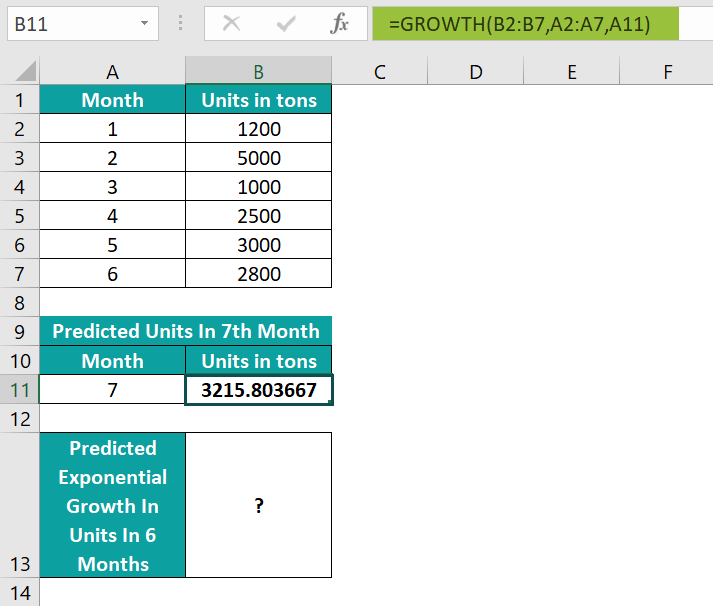Here, the formula accepts cell ranges B2:B7 and A2:A7 as the known y and x values, and cell A11 as the argument new_xs, and returns the estimated exponential growth in the 7th month, 3215.803667.

[Alternatively, we can select cell B11 → click FormulasMore FunctionsStatisticalGROWTH to open the Function Arguments window.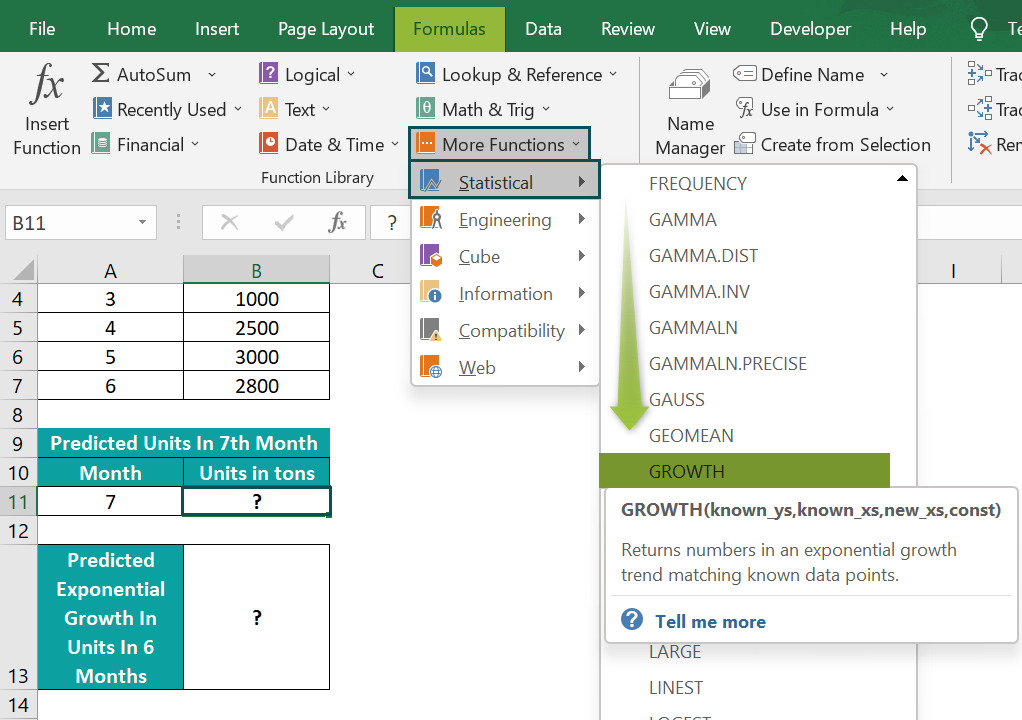Next, enter the required GROWTH() arguments in the Function Arguments window.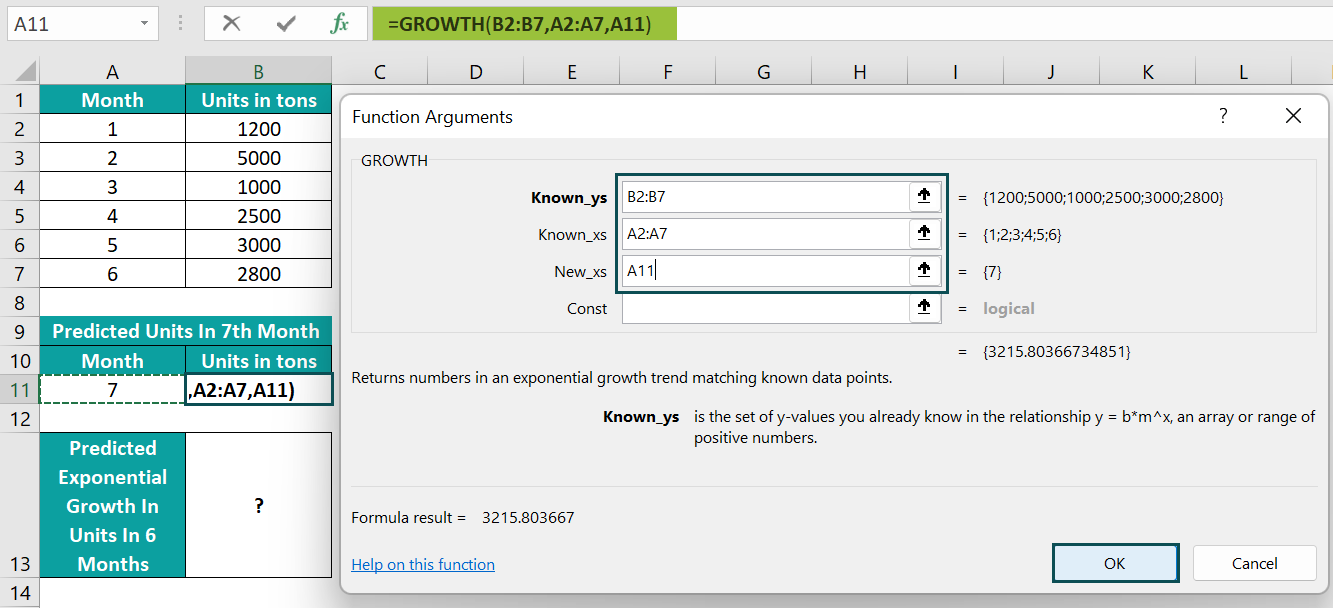And once we click OK in the Function Arguments window, the output is executed in cell B11.]

2. Then, select cell B13, enter the formula =GROWTH(B2:B7,A2:A7), and press Enter.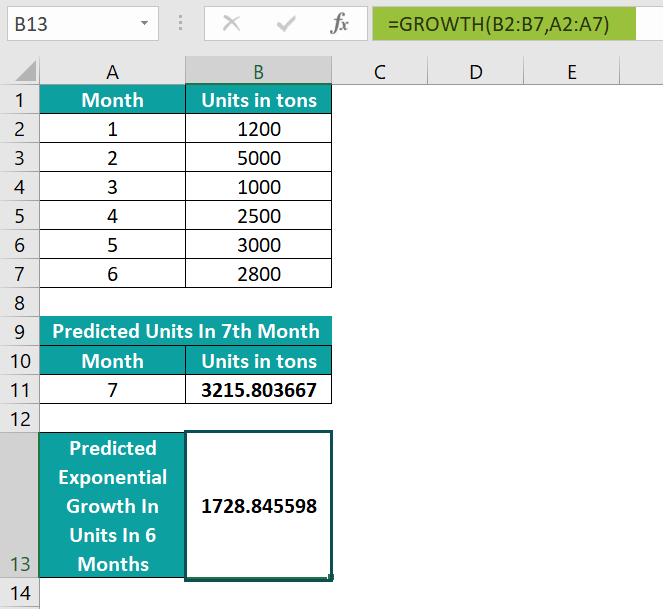Therefore, the formula accepts the cell ranges B2:B7 and A2:A7 as the known y and x values and returns the growth in the given six months as 1728.845598.

### Examples

We will understand some advanced scenarios using the GROWTH Excel formula examples.

#### Example #1

We can apply the GROWTH Excel function as an array formula to find the prediction results (new y-values) for more than one day (new x-values).

The following table shows the count of the orders dispatched in six days.

The procedure to predict the possible number of orders we can dispatch in the next four days (7, 8, 9, and 10) are,

Step 1: Select cell range B8:B11, enter the formula =GROWTH(B2:B7,A2:A7,A8:A11), and press the Ctrl + Shift + Enter keys to execute it as an array formula.

Step 2: And once we press the keys Ctrl + Shift + Enter, the array formula gets executed.

We get the output as shown above, the function returns the achievable number of orders we can dispatch in the required four days.

#### Example #2

We will predict the possible distance the car can cover in 11 hours using the ROUND excel function and the GROWTH formula.

The below table shows the distances a car covers in the respective hours.

The procedure to apply the GROWTH Excel function and the ROUND() function to predict the 11 hours distance are,

Step 1: Select cell B8, enter the formula =ROUND(GROWTH(B2:B7,A2:A7,A8),0), and press Enter.

We get the output as shown above.

[Output Observation: The formula accepts the cell ranges B2:B7 and A2:A7 as the known_ys and known_xs arguments, and the cell A8 value as the new_xs argument. And it returns the value 764.808633137768 miles as the predicted distance the car can travel in 11 hours.

The ROUND() then takes the value 764.808633137768 and 0 as the inputs to round off the number to zero decimal places, thus returning the value 765 miles as the final output.]

#### Example #3

Let us see how the GROWTH Excel function works along the lines of the below equation.

y = b * m^x

The given data includes the b value as 10 and the m value as 2, as depicted in the below image.

The steps to determine the new y-values for the new x-values using the given formula and GROWTH Excel function in the cell ranges B16:B20 and E16:E20, are as follows:

Step 1: Select cell B16, enter the equation formula =\$B\$3*\$B\$4^A16, and press Enter.

Step 2: Drag the formula from cell B16 to B20 using the fill handle.

Step 3: Select cell range E16:E20, and enter the formula =GROWTH(B8:B12,A8:A12,D16:D20,

Step 4: In continuation with the formula, Excel provides a drop-down list to pick the const argument. Double-click the required option, here, we choose TRUE, as we need to use the value b normally.

Step 5: Close the parenthesis and press Ctrl + Shift + Enter. It will get executed as an array formula.

We get the output as shown above. The given exponential formula and the GROWTH() results are the same.

### Important Things to Note

• Suppose the arrays, known_xs, and known_ys, have different lengths. Then the GROWTH Excel function output will be the #REF! error.
• If the supplied known_ys array includes values <=0, we will get the #NUM! error.
• For non-numeric known_xs, known_ys, or new_xs argument values, the output will be the #VALUE! error.

1. How to use GROWTH() to create an exponential graph in Excel?

We can use GROWTH() to create an exponential graph in Excel by first determining the y-values for the series of new x-values, and then create a Scatter plot graph.

For example, the table below contains arrays of known x- and y-values in cells A2:B6.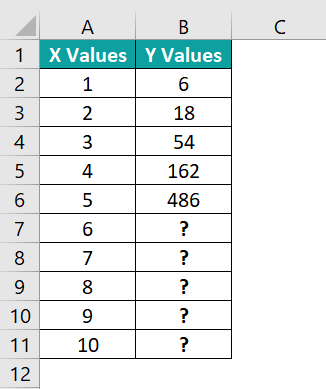The steps to plot an exponential graph for the entire dataset are as follows:

• 1: Select cells B7:B11, and enter the formula =GROWTH(B2:B6,A2:A6,A7:A11).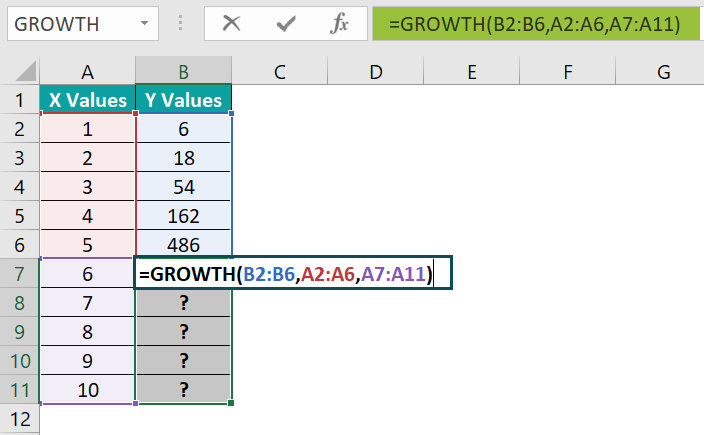• 2: Press Ctrl + Shift + Enter keys to execute the formula as an array formula.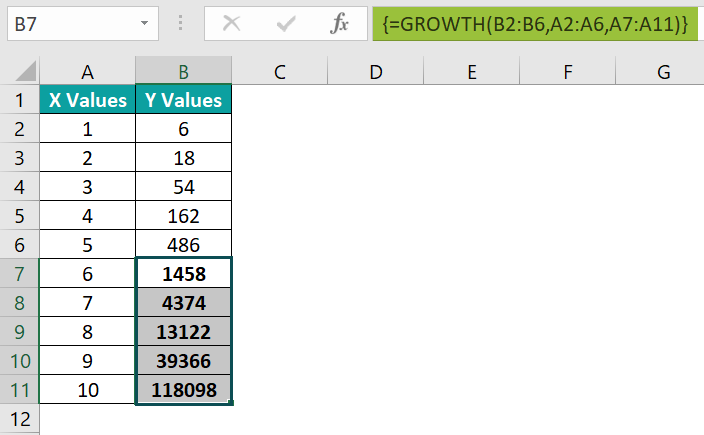• 3: Choose cell range A1:B11 → select the “Insert” tab → go to the “Charts” group → click the “Insert Scatter(X, Y) or Bubble chart” option drop-down → select the “Scatter” chart, as shown.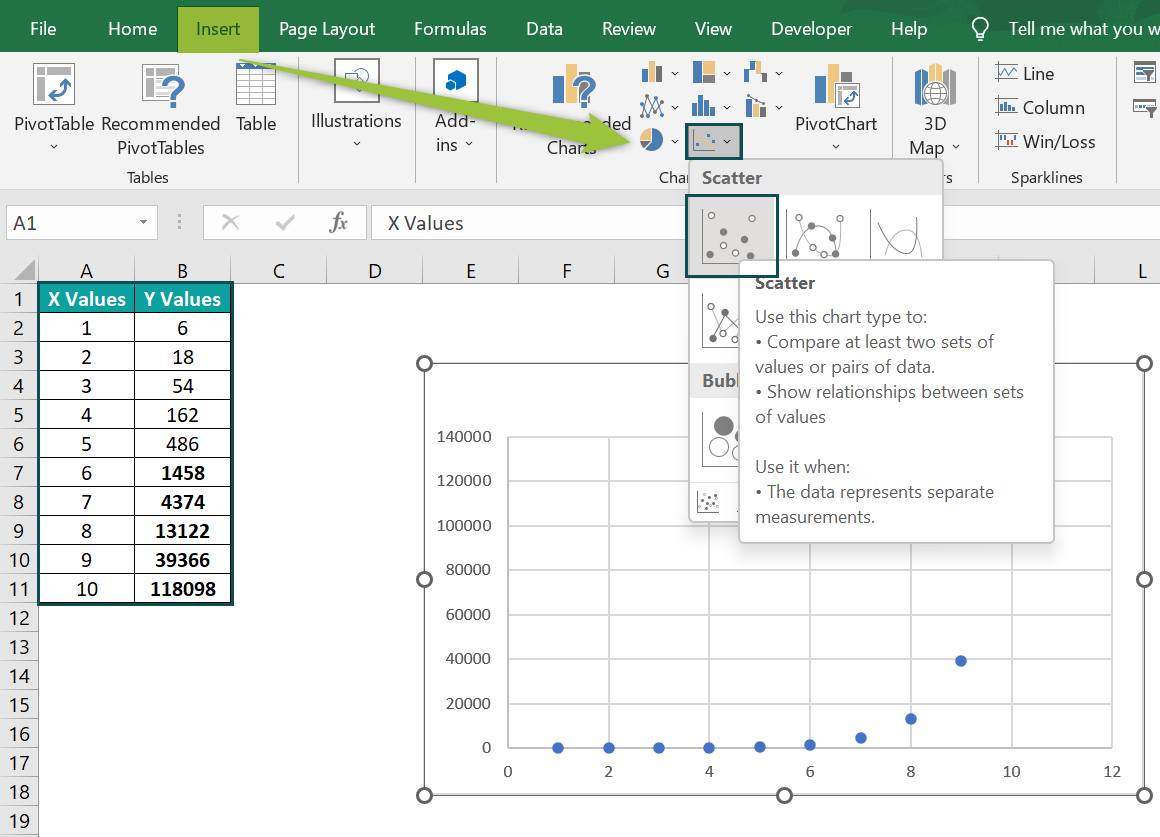• 4: Right-click on a data point in the plot area and select the Add Trendline option from the format menu to open the Format Trendline pane.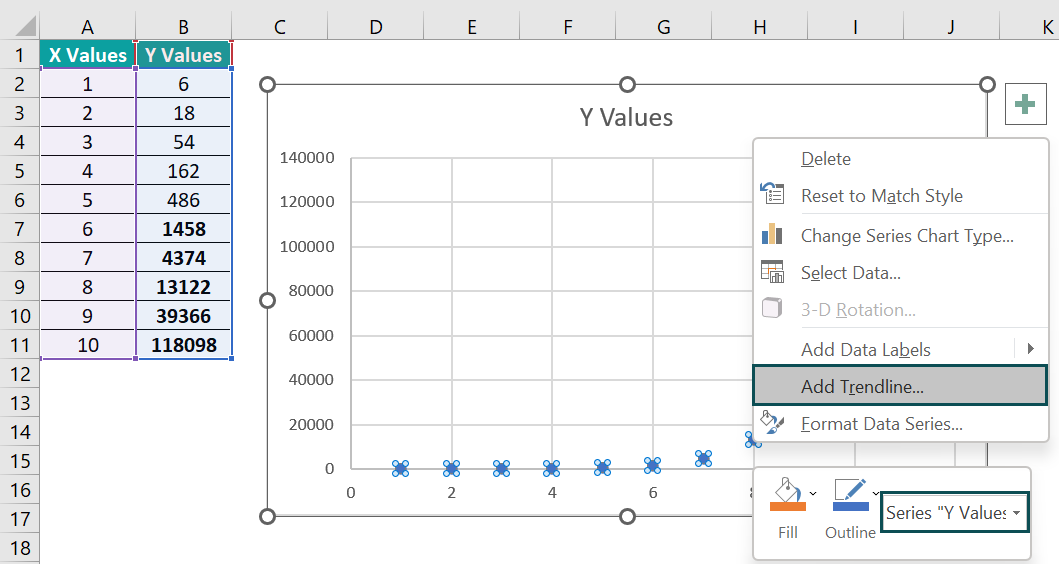The Trendline appears in the chart, and the “Format Trendline” window pane opens on the right side.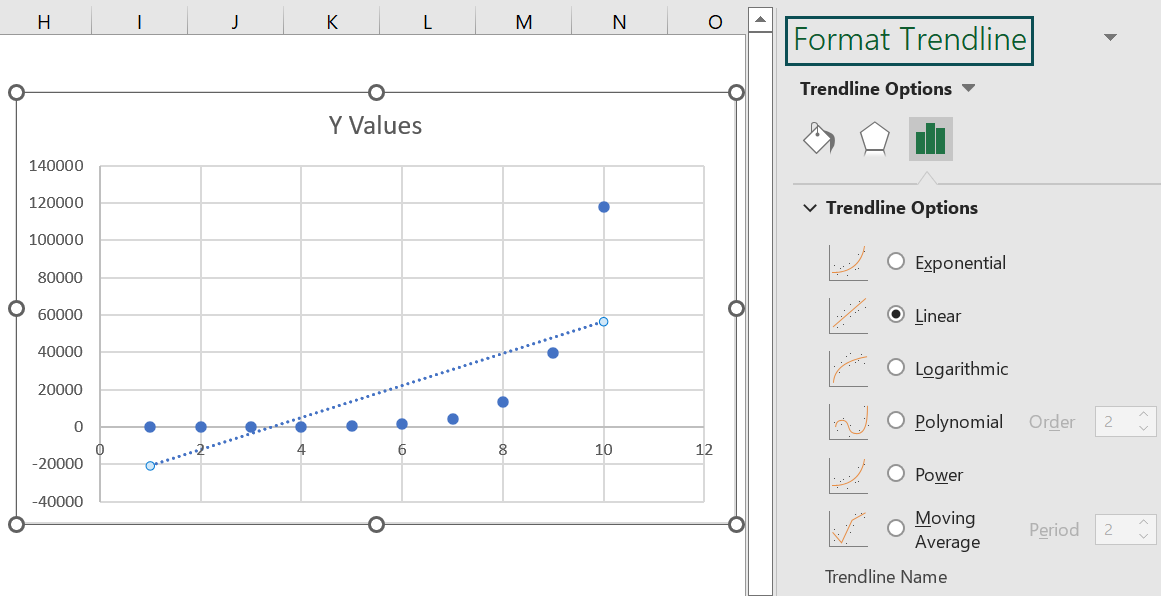• 5: Select the option Exponential from the Trendline Options and close the pane.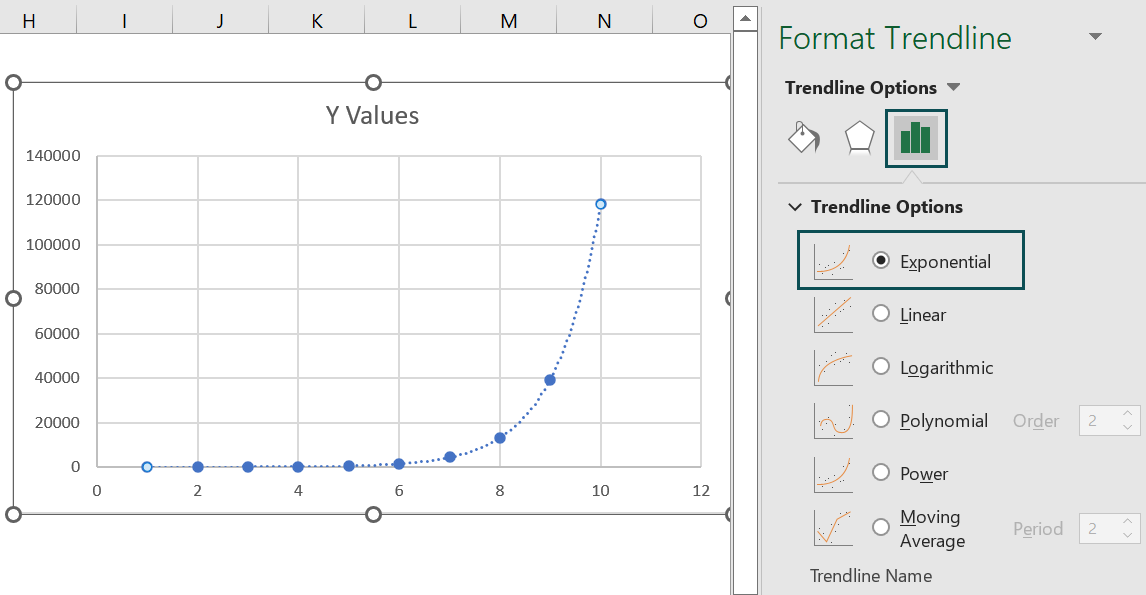• 6: Finally, using the Chart Elements option (‘+’ icon), enable the Axis Titles option and update the chart title and axes titles in the chart area to achieve the required exponential graph.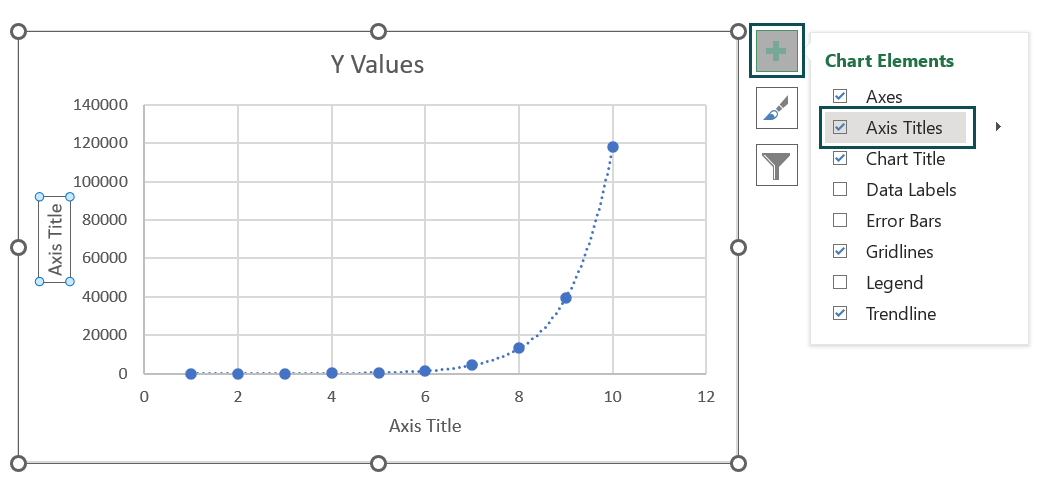Here, the final scatter graph output is shown below.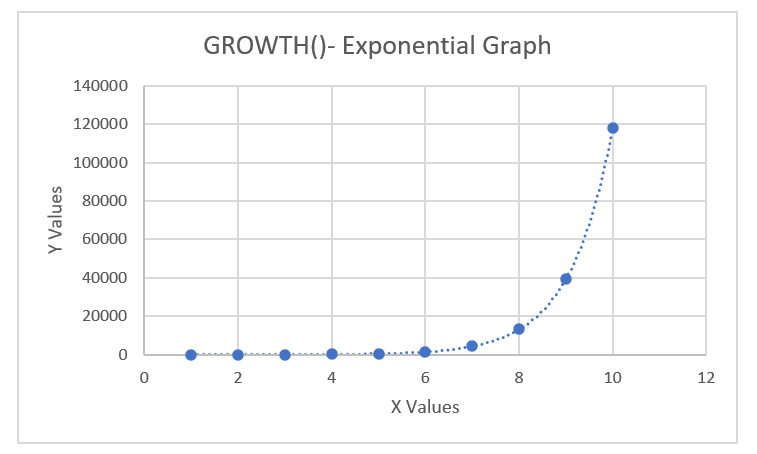2. What is the method to use GROWTH Excel formula?

We can use the GROWTH Excel formula as follows:

First, check if the source data does not contain any non-numeric argument values and zeros in the known_ys array. And ensure when the source data includes the known_xs array, the array length should be the same as that ofthe known_ys array.

1)    Select an empty cell for the output.
2)    Type =GROWTH( in the cell. [Alternatively, type =G and double-click the GROWTH function from the Excel suggestions.]
3)    Enter the arguments as cell values or cell references and close the brackets.
4)    Finally, press Enter to view the predicted exponential growth value.

3. Why is the GROWTH function in Excel not working?

The GROWTH function in Excel is not working, perhaps because of the following reasons:

The requirement is to determine y-values for an array of x-values. But we do not execute the GROWTH() as an array formula.
If the supplied known_xs and known_ys arrays have different lengths.
The entered known_ys array contains values less than or equal to 0.
If supplied known_xs, known_ys, or new_xs argument values are non-numeric.